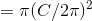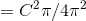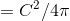## Example Questions

### Example Question #1 : How To Find The Ratio Of Diameter And Circumference

Letrepresent the area of a circle andrepresent its circumference. Which of the following equations expressesin terms of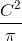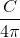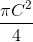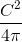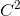Explanation:

The formula for the area of a circle is, and the formula for circumference is. If we solve for C in terms of r, we get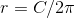.

We can then substitute this value of r into the formula for the area: